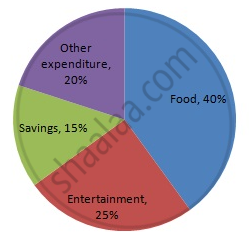Share

# The Following Table Shows How a Student Spends His Pocket Money During the Course of a Month. Represent It by a Pie-diagram. - Mathematics

Course
ConceptCircle Graph Or Pie Chart - Drawing Pie Charts

#### Question

The following table shows how a student spends his pocket money during the course of a month. Represent it by a pie-diagram.

 Items Food Entertainment Other expenditure Savings Expenditure 40% 25% 20% 15%

#### Solution

We know:
Central angle of a component = (component value/sum of component values x 360)
Here, total expenditure = 100%
Thus, central angle for each component can be calculated as follows:

 Item Expenditure(in %) Sector angles Food 40 40/100 x 360 = 144 Entertainment 25 25/100 x 360 = 90 Other expenditures 20 20/100 x 360 = 72 Savings 15 15/100 x 360 = 54

Now, the pie chat representing the given data can be constructed by following the steps below:
Step 1 : Draw circle of an appropriate radius.
Step 2 : Draw a vertical radius of the circle drawn in step 1.
Step 3 : Choose the largest central angle. Here the largest central angle is 144o. Draw a sector with the central angle 144o in such a way that one of its radii coincides with the radius drawn in step 2 and another radius is in its counter clockwise direction.
Step 4 : Construct other sectors representing the other items in the clockwise sense in descending order of magnitudes of their central angles.
​Step 5 : Shade the sectors with different colours and label them, as shown as in figure below.​​Is there an error in this question or solution?

#### APPEARS IN

RD Sharma Solution for Mathematics for Class 8 by R D Sharma (2019-2020 Session) (2017 to Current)
Chapter 25: Data Handling-III (Pictorial Representation of Data as Pie Charts or Circle Graphs)
Ex. 25.1 | Q: 11 | Page no. 13

#### Video TutorialsVIEW ALL 

Solution The Following Table Shows How a Student Spends His Pocket Money During the Course of a Month. Represent It by a Pie-diagram. Concept: Circle Graph Or Pie Chart - Drawing Pie Charts.
S